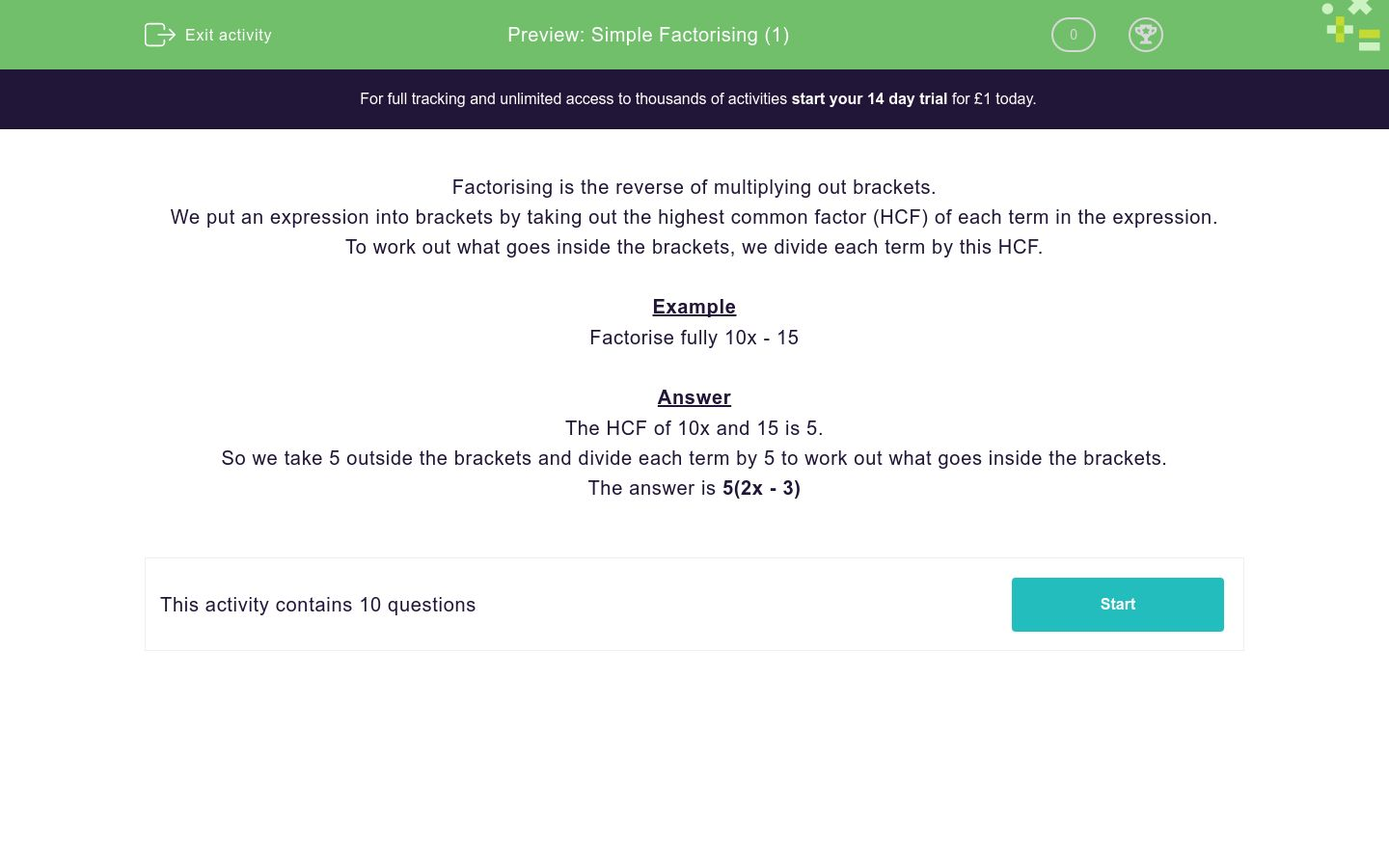# Simple Factorising (1)

In this worksheet, students factorise simple algebraic expressions.Key stage:  KS 3

Curriculum topic:   Algebra

Curriculum subtopic:   Understand Expressions, Equations, Inequalities, Terms and Factors

Difficulty level:### QUESTION 1 of 10

Factorising is the reverse of multiplying out brackets.

We put an expression into brackets by taking out the highest common factor (HCF) of each term in the expression.

To work out what goes inside the brackets, we divide each term by this HCF.

Example

Factorise fully 10x - 15

The HCF of 10x and 15 is 5.

So we take 5 outside the brackets and divide each term by 5 to work out what goes inside the brackets.

The answer is 5(2x - 3)

Factorise fully:

15x + 10

25x

5(3x + 2)

5(3x + 10)

Factorise fully:

15x + 5

3x + 1

5(3x + 5)

5(3x + 1)

Factorise fully:

15x - 25

3x - 1

5(3x - 5)

5(3x + 5)

Factorise fully:

9x - 6

3(3x - 2)

3(3x - 6)

9(x - 6)

Factorise fully:

9x - 18

3(3x - 2)

3(3x - 6)

9(x - 2)

Factorise fully:

27x - 6

3(9x - 2)

3(9x - 6)

9(3x - 6)

Factorise fully:

8x - 6

4(2x - 6)

4(x - 6)

2(4x - 3)

Factorise fully:

18x - 6

6(3x - 0)

6(3x -   )

6(3x - 1)

Factorise fully:

28y + 21

7y(4 + 3)

21(7y + 0)

7(4y + 3)

Factorise fully:

28y + 20

7y(4 + 13)

4(7y + 5)

7(4y + 13)

• Question 1

Factorise fully:

15x + 10

5(3x + 2)
EDDIE SAYS
The HCF (Highest Common Factor) here is 5 Firstly, we take the 5 and put it outside the bracket Secondly, we divide both terms by 5 to find out what goes inside the bracket This gives us 5(3x + 2)
• Question 2

Factorise fully:

15x + 5

5(3x + 1)
EDDIE SAYS
The HCF (Highest Common Factor) here is 5 Firstly, we take the 5 and put it outside the bracket Secondly, we divide both terms by 5 to find out what goes inside the bracket This gives us 5(3x + 1)
• Question 3

Factorise fully:

15x - 25

5(3x - 5)
EDDIE SAYS
The HCF (Highest Common Factor) here is 5 Firstly, we take the 5 and put it outside the bracket Secondly, we divide both terms by 5 to find out what goes inside the bracket This gives us 5(3x - 5) Don't worry that this one has a minus in the middle, we still do it the same way.
• Question 4

Factorise fully:

9x - 6

3(3x - 2)
EDDIE SAYS
The HCF (Highest Common Factor) here is 3 Firstly, we take the 3 and put it outside the bracket Secondly, we divide both terms by 3 to find out what goes inside the bracket This gives us 3(3x - 2)
• Question 5

Factorise fully:

9x - 18

9(x - 2)
EDDIE SAYS
The HCF (Highest Common Factor) here is 9 Firstly, we take the 9 and put it outside the bracket Secondly, we divide both terms by 9 to find out what goes inside the bracket This gives us 9(x - 2) The common mistake people make here is to say the answer is 3(3x-6) 3(3x-6) is not FULLY factorised as 3 is not the HIGHEST common factor
• Question 6

Factorise fully:

27x - 6

3(9x - 2)
EDDIE SAYS
The HCF (Highest Common Factor) here is 3 Firstly, we take the 3and put it outside the bracket Secondly, we divide both terms by 3 to find out what goes inside the bracket This gives us 3(9x - 2)
• Question 7

Factorise fully:

8x - 6

2(4x - 3)
EDDIE SAYS
The HCF (Highest Common Factor) here is 2 Firstly, we take the 2 and put it outside the bracket Secondly, we divide both terms by 2 to find out what goes inside the bracket This gives us 2(4x - 3)
• Question 8

Factorise fully:

18x - 6

6(3x - 1)
EDDIE SAYS
The HCF (Highest Common Factor) here is 6 Firstly, we take the 6 and put it outside the bracket Secondly, we divide both terms by 6 to find out what goes inside the bracket This gives us 6(3x - 1)
• Question 9

Factorise fully:

28y + 21

7(4y + 3)
EDDIE SAYS
The HCF (Highest Common Factor) here is 7 Firstly, we take the 7 and put it outside the bracket Secondly, we divide both terms by 7 to find out what goes inside the bracket This gives us 7(4y + 3)
• Question 10

Factorise fully:

28y + 20

4(7y + 5)
EDDIE SAYS
The HCF (Highest Common Factor) here is 4 Firstly, we take the 4 and put it outside the bracket Secondly, we divide both terms by 4 to find out what goes inside the bracket This gives us 4(7y + 5)
---- OR ----

Sign up for a £1 trial so you can track and measure your child's progress on this activity.

### What is EdPlace?

We're your National Curriculum aligned online education content provider helping each child succeed in English, maths and science from year 1 to GCSE. With an EdPlace account you’ll be able to track and measure progress, helping each child achieve their best. We build confidence and attainment by personalising each child’s learning at a level that suits them.

Get started